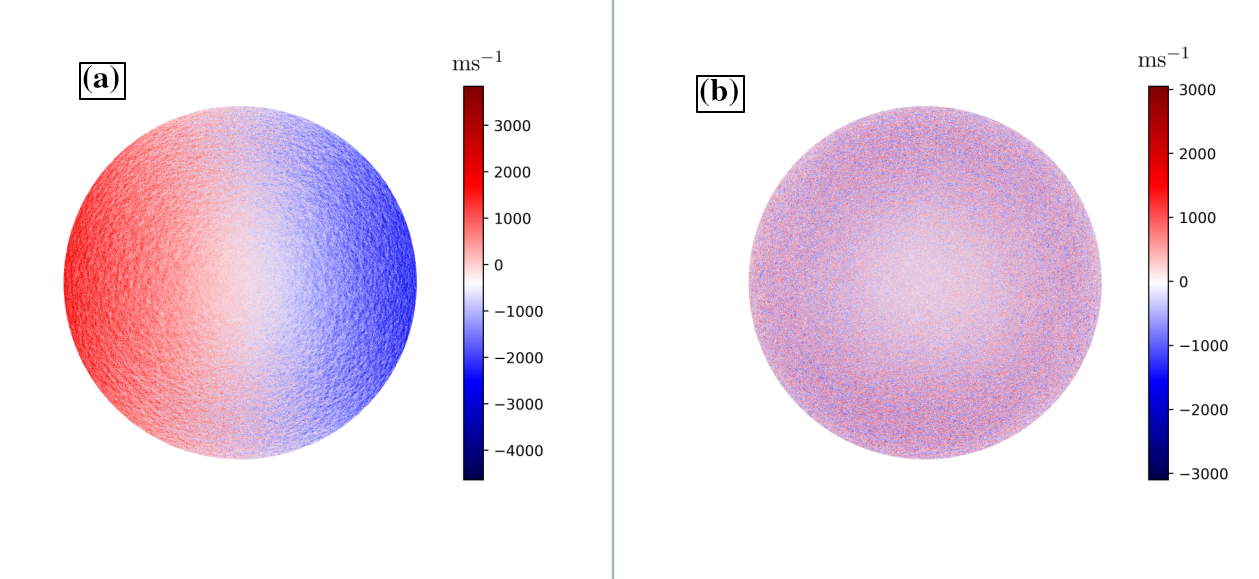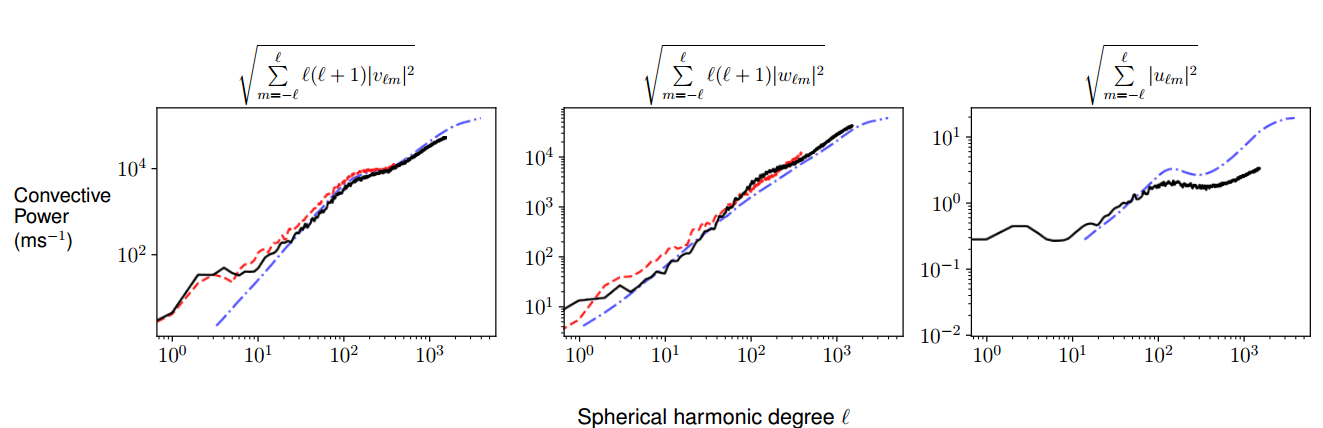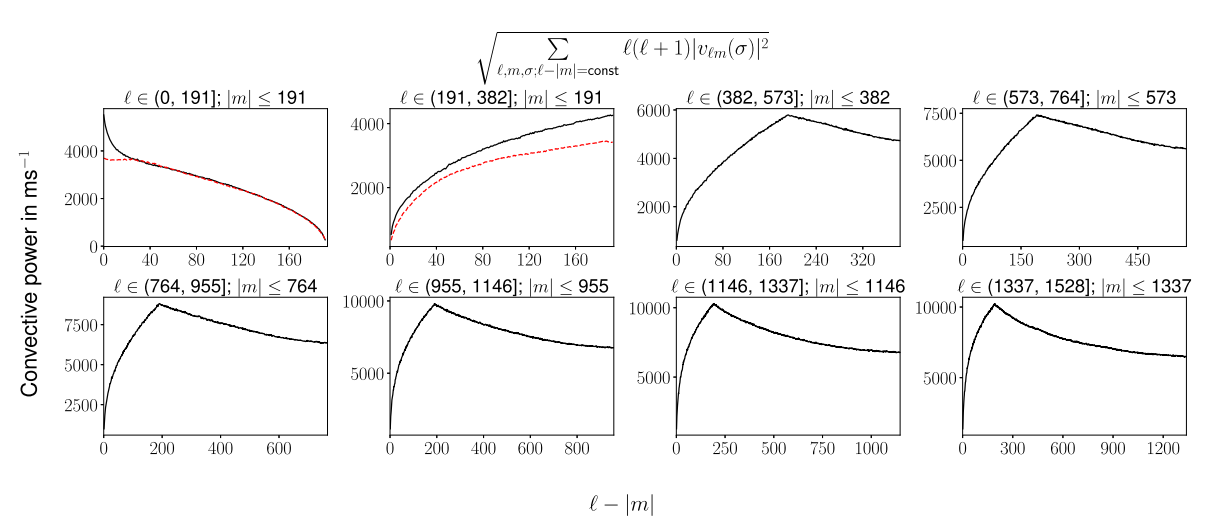# 164. Characterizing solar surface convection using Doppler measurements

Contributed by Samarth G. Kashyap. Posted on September 29, 2021

Samarth G. Kashyap
Department of Astronomy and Astrophysics, Tata Institute of Fundamental Research, Mumbai-400005, India

Coherent Structure Tracking (CST) analysis of solar granules has been shown to be a reliable method to obtain the horizontal convection spectrum by Rincon et. al. . However, the resolution of the inferred velocity map is limited by the size of the structure tracked (granule). We have developed a new method to characterize the multiscale convective spectrum of the Sun using the high-resolution line-of-sight Dopplergram images from HMI. This method enables us to estimate the spectrum to the finest observable scales. In the present work, we obtain the spectrum up to$\ell$=1535. We also use Heirarchical Equal Area Pixelization (HEALPix) scheme for spectral analysis, which enables a high-speed computation of spherical harmonic transforms.

Before processing the data, we remove features that affect the inference of the surface convection spectrum. We setup a pipeline to clean the raw images from HMI. The features are listed below.

1. Motion of the Observer – Since Doppler measurements observe the relative velocity between the observer and the source, the motion of the observer needs to be compensated for. The velocity of SDO in the frame of the Sun is provided through the ‘OBS_VR’, ‘OBS_VN’, ‘OBS_VW’ parameters of the HMI Dopplergram FITS file.
2. Gravitational Redshift – The solar surface is at a higher gravitational potential than the HMI.
3. Convective Blueshift – Unresolved granules on the solar disk gives rise to an apparent blueshift when moving from the disk-center to the limb.
4. Rotation and Meridional circulation – Large scale flows that contribute to the Doppler measurements.
5. Imaging artifacts – Artifacts which are fixed in the image – obtained by averaging over multiple images after the removal of features (1-4).

The pipeline for processing of raw HMI images can be found at [here] and processed image is shown in Figure 1.Figure 1| Dopplergram images are shown here. Panel (a) shows the raw image obtained from HMI. The dominant feature of rotation is clearly visible. Panel (b) shows the image after rotation, meridional circulation, limb-shift, gravitational redshift and imaging artefacts are removed.

The Doppler velocity on the surface can be expanded in the vector spherical harmonic basis.$\tilde{\bf{u}}(\theta, \phi) = \sum_{\ell m}\tilde{u}_{\ell m} Y_{\ell m}(\theta, \phi) \hat{\bf{r}}+ \tilde{v}_{\ell m} \mathbf{\nabla}_hY_{\ell m}(\theta, \phi) + \tilde{w}_{\ell m} \hat{\bf{r}}\times \mathbf{\nabla}_hY_{\ell m}(\theta, \phi)$,

where$Y_{\ell m}$ are the spherical harmonics and$\mathbf{\nabla}_h$ is the horizontal gradient operator given by$\mathbf{\nabla}_h = \hat{\theta}\frac{\partial}{\partial \theta} + \hat{\phi}\frac{1}{\sin\theta}\frac{\partial}{\partial \phi}$. The observed Doppler velocity corresponds to the line-of-sight component on roughly half the solar surface i.e.$\mathbf{u}(\theta, \phi) = [\hat{l}(\theta, \phi)\cdot\tilde{\mathbf{u}}(\theta, \phi)] \, \hat{l}(\theta, \phi)$. Hence the observed spectral components$\mathcal{U} = (u_{\ell m}, v_{\ell m}, w_{\ell m})$ are not the same as the true spectral components$\tilde{\mathcal{U}} = (\tilde{u}_{\ell m}, \tilde{v}_{\ell m}, \tilde{w}_{\ell m})$. We show that these components are linearly related ($\mathcal{U} = \mathcal{M}\tilde{\mathcal{U}}$) through the mixing matrix$\mathcal{M}$. The observed spectrum$\tilde{\mathcal{U}}$ is obtained using HEALPix, and the “true” spectrum is computed through the inversion of the mixing matrix$\mathcal{M}$. This inversion procedure has been comprehensively tested for various synthetic spectral profiles, and it is found that poloidal flow recovery is the most reliable. Figure 2 shows a comparison of the inverted spectrum with other methods.Figure 2| A comparison of convective power spectra from 3 different methods. The panels show poloidal, toroidal and radial spectrum. The black line shows the current work, the red dashed line shows the spectrum obtained using Local Correlation Tracking (LCT; since only horizontal velocities are obtained, the red LCT spectrum does not provide a spectrum for radial velocity). The blue dashdot line shows the spectrum obtained by Hathaway.

Obtaining the complete$\ell, m$ spectrum enables us to study whether the spectrum is dominated by zonal, sectoral or tesseral harmonics. Figure 3 shows the spectrum plotted as a function of$\ell - |m|$. The poloidal spectrum appears to be sectorally dominant, i.e., a strong peak at low$\ell - |m|$, which indicates the variation of convective power as a function of latitude.Figure 3| Poloidal flow spectra are computed for different length scales.For each range of$\ell$, the spectrum is plotted as a function of$\ell - |m|$.The spectrum obtained from LCT is shown as red-dashed line. For the lowest$\ell$ a strong peak is seen in the present work which is not seen in the LCT data.

For more details of this work, please refer to Ref. .

### References:

 Rincon, F., Roudier, T., Schekochihin, A. A. & Rieutord, M. 2017, A&A, 599 A69
 Górski, K. M., Hivon, E., Banday, A. J., Wandelt, B. D., Hansen, F. K. Reinecke, M. & Bartelmann, M. 2005, ApJ, 622, 759
 Hathaway, David H., Teil, Thibaud, Norton, Aimee A. & Kitiashvili, Irina, 2015, ApJ, 811, 105
 Kashyap, Samarth G. & Hanasoge, Shravan M. 2021, ApJ, 916, 87